# 5.1 Calculation of Kcouple with mode decomposition

The introduction of wires into a rectangular enclosure by a multi-mode analogous transmission line theory has been presented by  and . The introduction of traces to the parallel-plane cavity field formulations in Chapter 4 can be achieved by mode decomposition . The cover plane return current is identified as the parasitic common mode current depicted in Figure 5.3.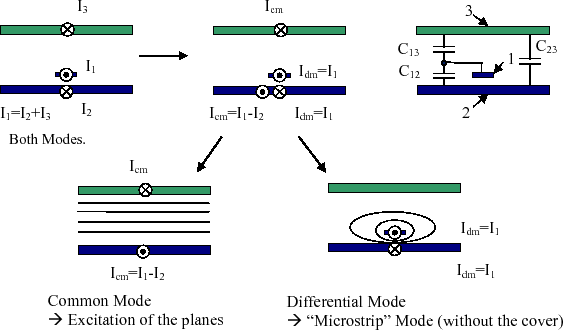Figure 5.3: Identification of the cover plane return current as the parasitic common mode current.

The conductor with number 1 in Figure 5.3 is the trace, the ground plane is assigned to number 2 and the cover plane to number 3. The partial capacitances between these conductors are indexed accordingly. A source which drives the traces against the ground plane will excite both, the differential mode and the common mode currents. The partial capacitance between the cover and the ground plane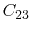is high, due to the large extent of these planes. A source current that drives the trace is divided by the capacitancesand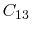. Therefore, the excitation of the cavity field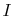in (4.13) by the trace is expressed by the trace currents multiplied by the coupling factor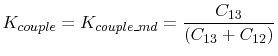(5.7)

To extract the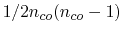partial capacitances between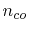conductors, the Laplace equation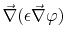with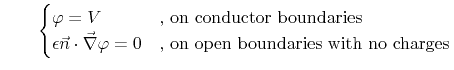(5.8)

for the electrostatic potential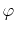has to be solved for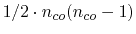different voltage distributions , . The surface normal vector at the boundary is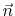. The Smart Analysis Program (SAP), a FEM based interconnect simulation software from , performs this partial capacitance extraction automatically. SAP is also capable of automated resistance and inductance extraction of interconnects.
Figure 5.4 depicts the difference in the electrostatic potential distribution between a trace with and without cover plane.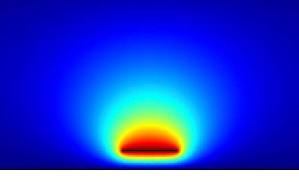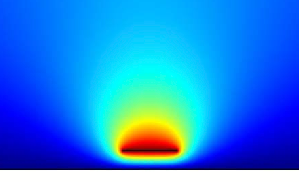(a) Field with cover plane. (b) Field without cover plane.
Figure 5.4: Electrostatic potential with and without the metallic cover plane (qualitative diagram).

C. Poschalko: The Simulation of Emission from Printed Circuit Boards under a Metallic Cover